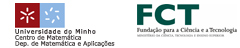EN || PT || ESPaulo Amorim (CMAF e Universidade de Lisboa)# We prove convergence of some numerical schemes for short wave long wave interaction equations. These are systems of nonlinear PDEs consisting of a Schroedinger equation for the short waves and either a KdV equation or a nonlinear conservation law modeling the long waves. We present some computations and a numerical study of some open problems. This is joint work with M. Figueira of CMAF-UL.© 3º Encontro Ibérico de Matemática :: 2010